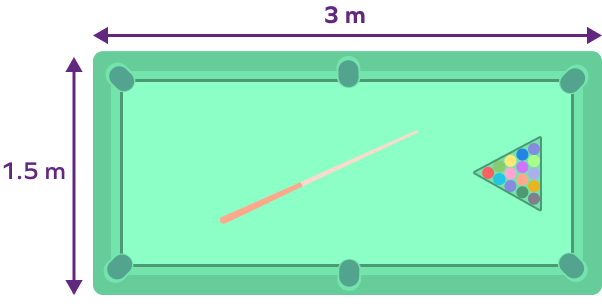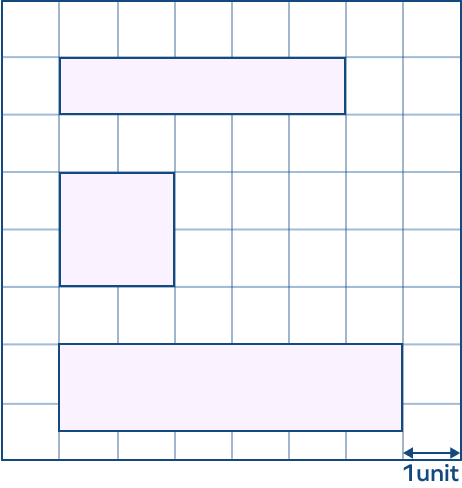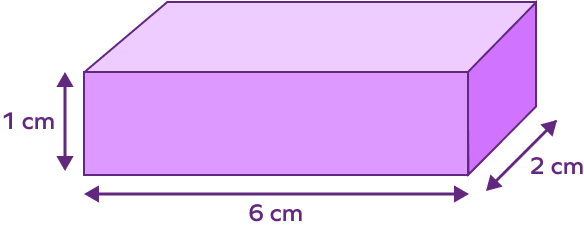# Width – Definition with Examples

## What Is Width in Math?

All the living and nonliving things around us are of different shapes and sizes. We can describe their size in various ways. One of them is by measuring their width. What does width mean in geometry? The measure of an object from side to side is known as width in math.

The given image shows us the width of a swimming pool.

## Width Definition

Width can be defined as the horizontal measurement or distance measured from side to side.

Which way is width measured? It is measured at right angles to the length. Width measures an object from one side to another. For example, we tell how wide a road is by measuring the distance from one side of the road to the other.

## Difference between Width and Length

“Width” in geometry is often used to describe the shorter side of objects. For example, in the given rectangles, both are the same rectangle, but one is rotated just to show that the shorter side of the rectangle is called the width.

The term “length” is often used alongside width in geometry to describe the longer of the lengths in an object.

Let us understand width better with an everyday example. Look at the image of a door, the shorter measurement of the door is its width. It is indicated by the double arrow and is also the measure of the door from side to side.

Width can be measured in standard units like meter, centimeter, millimeter.

Other customary units are inch, yard, foot.

## Difference between Width and Depth

Is width the same as depth? What is the difference between these measurements? Let’s see.

In two-dimensional shapes, width is the shortest side and tells us how broad an object is. Some objects around you, which have width, are book, wall, door, black board, desk, etc.

Depth is used to tell us how deep a three-dimensional object is. It is the side perpendicular to length and width.

We use the word depth when we talk about objects that are under the ground. Some things that have depth include well, pit, lake etc,.

## Width of Different Two-dimensional Shapes

In two-dimensional shapes, the width is the shortest side.

Let us now see how we represent width in different two-dimensional mathematical shapes.

Width of certain shapes are referred to with special names, for example, width of a rectangle is also known as its breadth.

In the case of a square, either of the sides can be width since all the sides of a square are equal.

## Width of Different Three-dimensional Shapes

In three-dimensional shapes, the shortest measure need not be the width. The side that represents width depends on the orientation of the shape.

Let us see the width of three-dimensional shapes.

## Conclusion

If you are tired of trying out dresses to see if they are your size, you can measure your body width and the width of your dress to check if they fit you.

## Solved Examples

1. Which eraser has the maximum width?

Solution:

Width is the side to side measure of an object. By counting the squares we can see that eraser A and B are less than 2 centimeters wide and eraser C is more than 2 centimeters wide.

Therefore, eraser C has the maximum width.

2. What is the width of the given rectangle?

Solution: The shorter side of the rectangle is the width. Therefore, the width of the rectangle is 5 cm.

3. What is the width of the given rectangle?

Solution:

By counting the squares along the width, we get the width of the rectangle as 2 units.

4. Which rectangle has the shortest width?

Solution: The shortest side of the rectangle is known as the length. By counting the squares, we can see that rectangle A has the least measurement of the shortest side. Hence, rectangle A has the shortest width.

5. Give the width of the cuboid shown.

Solution: The width of the cuboid is 1 cm.

## Practice Problems

1

### Look at the image of the pool table, what is its width?3 cm
1.5 cm
3 m
1.5 m
CorrectIncorrect
The width of the pool table is 1.5 m.
2

### Which rectangle has the greatest width?A
B
C
Both A and C
CorrectIncorrect
Rectangle B has the greatest width of 2 units.
3

### What is the width of the given rectangle?

5 cm
4 cm
3 cm
2 cm
CorrectIncorrect
Width of the rectangle is its shortest side, i.e., 4 cm.
4

### Give the width of the cuboid shown.6 cm
2 cm
1 cm
9 cm
CorrectIncorrect
Width of the cuboid is 2 cm.
5

### The _________ is the shortest side of a rectangle.

length
height
depth
width
CorrectIncorrect
The shortest side of any two-dimensional shape is known as its width, so the width is also the shortest side of the rectangle.

Yes, width measurement can be in decimals or fractions. It is the side to side distance of an object.

Hand span is a non-standard measurement. It is the width of the hand when the fingers are spread out. Yes, width can be measured by hand span.

We know that the formula to find area of rectangle is as follows

Width $\times$ Length $=$ Area of rectangle

So, we can find the width of the rectangle by dividing the area by the length of rectangle. Width $= \frac{Area of rectangle}{Length}$

Nowadays, we order so many items online. Observe the parcels you get. Sometimes, they may look like the image below where the width is greater than the height.

Here, width is not the shortest side.

Depth is when you measure an object from top to bottom, generally from surface to a level deeper than the surface. For example, the depth of a swimming pool, well, ocean, etc. Height is also measured from top to bottom but it is from surface to a level higher than the surface and tells us how high is an object or example, height of an airplane from the ground.